# 树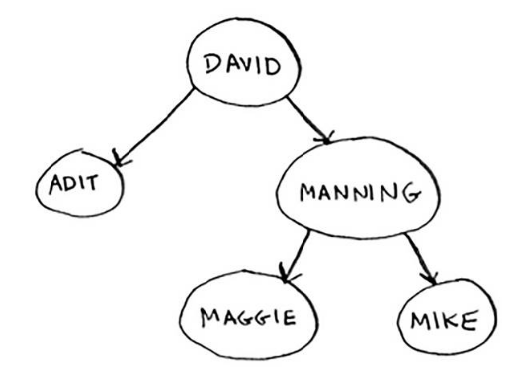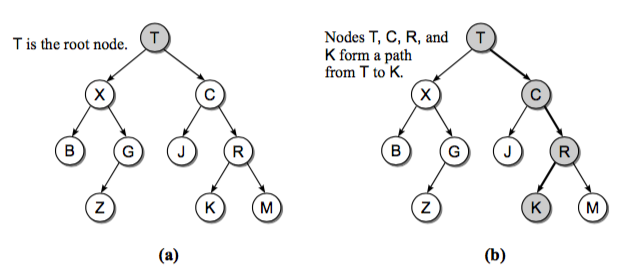• 根节点(root): 树的最上层的节点，任何非空的树都有一个节点
• 路径(path): 从起始节点到终止节点经历过的边
• 父亲(parent)：除了根节点，每个节点的上一层边连接的节点就是它的父亲(节点)
• 孩子(children): 每个节点由边指向的下一层节点
• 兄弟(siblings): 同一个父亲并且处在同一层的节点
• 子树(subtree): 每个节点包含它所有的后代组成的子树
• 叶子节点(leaf node): 没有孩子的节点成为叶子节点

# 二叉树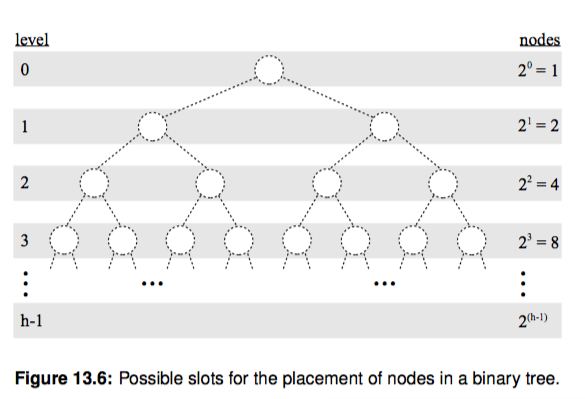• 节点深度(depth): 节点对应的 level 数字
• 树的高度(height): 二叉树的高度就是 level 数 + 1，因为 level 从 0开始计算的
• 树的宽度(width): 二叉树的宽度指的是包含最多节点的层级的节点数
• 树的 size：二叉树的节点总个数。

# 一些特殊的二叉树

### 满二叉树(full binary tree)### 完美二叉树(perfect binary tree)### 完全二叉树(complete binary tree)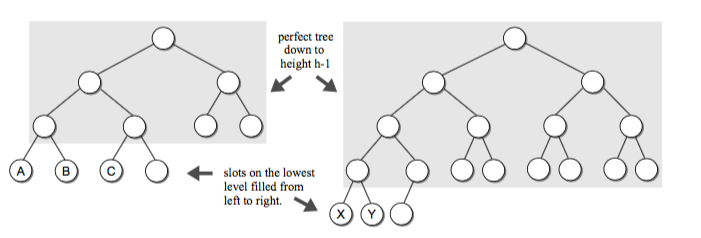# 二叉树的表示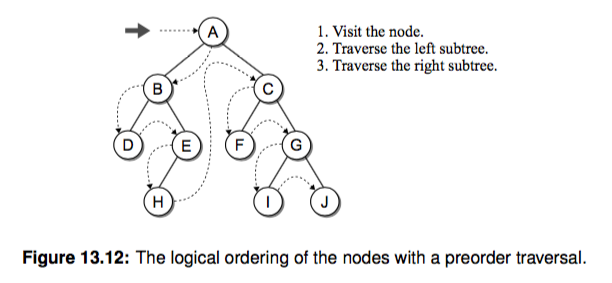class BinTreeNode(object):
def __init__(self, data, left=None, right=None):
self.data, self.left, self.right = data, left, right


class BinTree(object):
def __init__(self, root=None):
self.root = root


node_list = [
{'data': 'A', 'left': 'B', 'right': 'C', 'is_root': True},
{'data': 'B', 'left': 'D', 'right': 'E', 'is_root': False},
{'data': 'D', 'left': None, 'right': None, 'is_root': False},
{'data': 'E', 'left': 'H', 'right': None, 'is_root': False},
{'data': 'H', 'left': None, 'right': None, 'is_root': False},
{'data': 'C', 'left': 'F', 'right': 'G', 'is_root': False},
{'data': 'F', 'left': None, 'right': None, 'is_root': False},
{'data': 'G', 'left': 'I', 'right': 'J', 'is_root': False},
{'data': 'I', 'left': None, 'right': None, 'is_root': False},
{'data': 'J', 'left': None, 'right': None, 'is_root': False},
]


class BinTree(object):
def __init__(self, root=None):
self.root = root

@classmethod
def build_from(cls, node_list):
"""通过节点信息构造二叉树
第一次遍历我们构造 node 节点
第二次遍历我们给 root 和 孩子赋值
最后我们用 root 初始化这个类并返回一个对象

:param node_list: {'data': 'A', 'left': None, 'right': None, 'is_root': False}
"""
node_dict = {}
for node_data in node_list:
data = node_data['data']
node_dict[data] = BinTreeNode(data)
for node_data in node_list:
data = node_data['data']
node = node_dict[data]
if node_data['is_root']:
root = node
node.left = node_dict.get(node_data['left'])
node.right = node_dict.get(node_data['right'])
return cls(root)
btree = BinTree.build_from(node_list)


# 二叉树的遍历

• 先(根)序遍历: 先处理根，之后是左子树，然后是右子树
• 中(根)序遍历: 先处理左子树，之后是根，最后是右子树
• 后(根)序遍历: 先处理左子树，之后是右子树，最后是根

class BinTreeNode(object):
def __init__(self, data, left=None, right=None):
self.data, self.left, self.right = data, left, right

class BinTree(object):
def __init__(self, root=None):
self.root = root

@classmethod
def build_from(cls, node_list):
"""通过节点信息构造二叉树
第一次遍历我们构造 node 节点
第二次遍历我们给 root 和 孩子赋值

:param node_list: {'data': 'A', 'left': None, 'right': None, 'is_root': False}
"""
node_dict = {}
for node_data in node_list:
data = node_data['data']
node_dict[data] = BinTreeNode(data)
for node_data in node_list:
data = node_data['data']
node = node_dict[data]
if node_data['is_root']:
root = node
node.left = node_dict.get(node_data['left'])
node.right = node_dict.get(node_data['right'])
return cls(root)

def preorder_trav(self, subtree):
""" 先(根)序遍历

:param subtree:
"""
if subtree is not None:
print(subtree.data)    # 递归函数里先处理根
self.preorder_trav(subtree.left)   # 递归处理左子树
self.preorder_trav(subtree.right)    # 递归处理右子树

node_list = [
{'data': 'A', 'left': 'B', 'right': 'C', 'is_root': True},
{'data': 'B', 'left': 'D', 'right': 'E', 'is_root': False},
{'data': 'D', 'left': None, 'right': None, 'is_root': False},
{'data': 'E', 'left': 'H', 'right': None, 'is_root': False},
{'data': 'H', 'left': None, 'right': None, 'is_root': False},
{'data': 'C', 'left': 'F', 'right': 'G', 'is_root': False},
{'data': 'F', 'left': None, 'right': None, 'is_root': False},
{'data': 'G', 'left': 'I', 'right': 'J', 'is_root': False},
{'data': 'I', 'left': None, 'right': None, 'is_root': False},
{'data': 'J', 'left': None, 'right': None, 'is_root': False},
]
btree = BinTree.build_from(node_list)
btree.preorder_trav(btree.root)    # 输出 A, B, D, E, H, C, F, G, I, J


# 二叉树层序遍历

    def layer_trav(self, subtree):
cur_nodes = [subtree]  # current layer nodes
next_nodes = []
while cur_nodes or next_nodes:
for node in cur_nodes:
print(node.data)
if node.left:
next_nodes.append(node.left)
if node.right:
next_nodes.append(node.right)
cur_nodes = next_nodes  # 继续遍历下一层
next_nodes = []


    def layer_trav_use_queue(self, subtree):
q = Queue()
q.append(subtree)
while not q.empty():
cur_node = q.pop()
print(cur_node.data)
if cur_node.left:
q.append(cur_node.left)
if cur_node.right:
q.append(cur_node.right)

from collections import deque
class Queue(object):  # 借助内置的 deque 我们可以迅速实现一个 Queue
def __init__(self):
self._items = deque()

def append(self, value):
return self._items.append(value)

def pop(self):
return self._items.popleft()

def empty(self):
return len(self._items) == 0


# 反转二叉树

    def reverse(self, subtree):
if subtree is not None:
subtree.left, subtree.right = subtree.right, subtree.left
self.reverse(subtree.left)
self.reverse(subtree.right)


# 练习题

• 请你完成二叉树的中序遍历和后序遍历以及单元测试
• 树的遍历我们用了 print，请你尝试换成一个 callback，这样就能自定义处理树节点的方式了。
• 请问树的遍历操作时间复杂度是多少？假设它的 size 是 n
• 你能用非递归的方式来实现树的遍历吗？我们知道计算机内部使用了 stack，如果我们自己模拟如何实现？请你尝试完成
• 只根据二叉树的中序遍历和后序遍历能否确定一棵二叉树？你可以举一个反例吗？